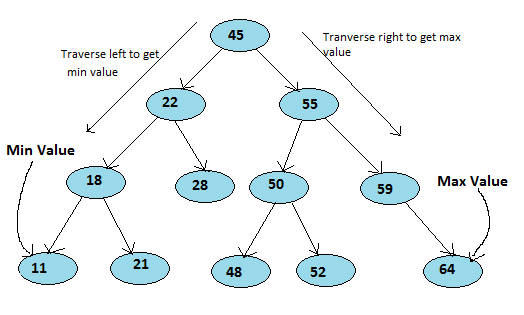## Friday, 2 March 2018

### Program to find maximum and minimum value from Binary Search Tree in Java

In previous post, discussed about to delete a node from Binary Search Tree. In this post, we will see how to find the max and min value of binary search tree.

Keep in mind :-- In a BST, the data of all the nodes in the left sub-tree of the root node should be less than or equals to the data of the root and the data of all the nodes in the right sub tree of the root node should be greater than the data of the root.

In this program, to find the min value just you can traverse a node to left to the end, Same thing for the max value also. Traverse a node to right to the end.

See the below BST,Minimum and Maximum value in BST

MaxMinBSTNode.java

```package com.test;

public class Node {

Integer data;
Node leftChild;
Node rightChild;

public Node(Integer data) {
this.data = data;
leftChild = null;
rightChild = null;
}
}

public class MaxMinBSTNode {

Node root;

private Integer getMaxNode() {
return getMaxNode(root);
}

private Integer getMinNode() {
return getMinNode(root);
}

private Integer getMaxNode(Node node) {

if (node.rightChild != null) {
return getMaxNode(node.rightChild);
}
return node.data;
}

private Integer getMinNode(Node node) {
if(node.leftChild != null) {
return getMinNode(node.leftChild);
}
return node.data;
}

public static void main(String[] args) {

MaxMinBSTNode tree = new MaxMinBSTNode();
tree.root = new Node(22);
tree.root.leftChild = new Node(10);
tree.root.rightChild = new Node(30);
tree.root.leftChild.leftChild = new Node(6);
tree.root.leftChild.rightChild = new Node(14);
tree.root.rightChild.leftChild = new Node(27);
tree.root.rightChild.rightChild = new Node(32);

System.out.println("Max value:--"+tree.getMaxNode());
System.out.println("Min value:--"+tree.getMinNode());
}
}
```

Output:-- Max value:-- 32
Min value:--6

Related Post:--
1) Java Program to delete a node from Binary Search Tree(BST)
2) Java Program to Count the number of nodes and leaf nodes of Binary Tree
3) Java Program to Reverse a Linked List using Recursion and Loops
4) How to Remove duplicates from ArrayList in Java
5) Java Program to Count Occurrence of Word in a Sentence